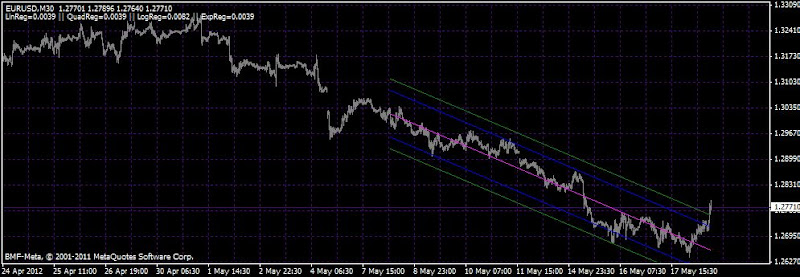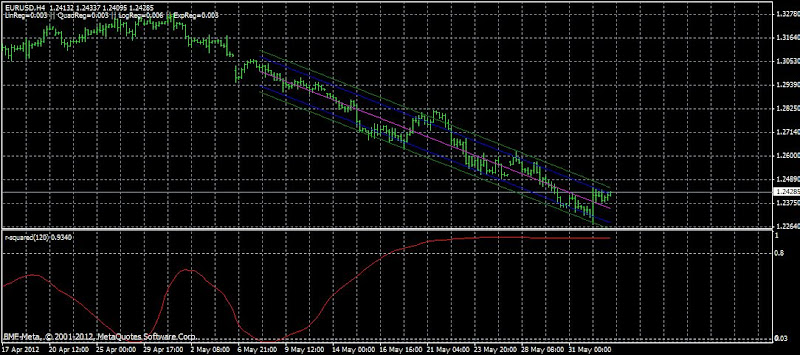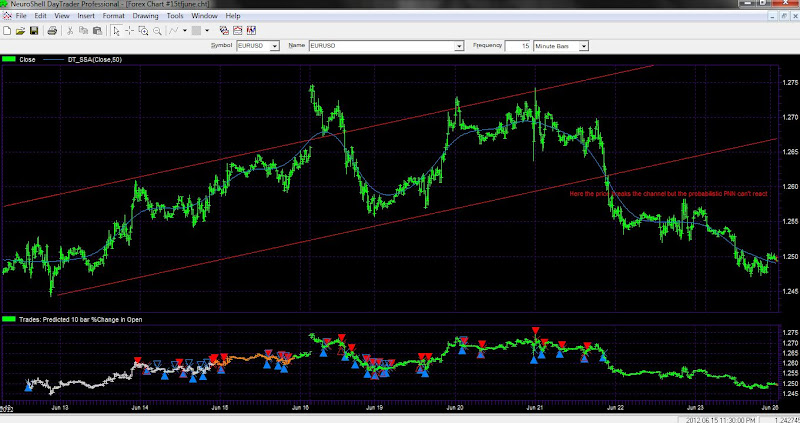## Regression Analysis Mod

• Public
By JohnLast 2929 days ago Comments (6)

This indicator compares four types of regression (linear, quadratic, logarithmic and exponential) and chooses the one that best fits the data analyzed.

This analysis is done every time there is a new tick.

In the left top corner of the chart four values are displayed. These values represent the dispersion of data regarding to its regression, so the smallest value indicates the best option. Additionally, the indicator allows recording these values into a text file for further analysis.

The source of this file is here.

What I did is to add another channel, so we have two channels instead of 1. The default values are 1.36 and 1.96 but you can use 2 and 3.От Indicators

• JohnLast 2929 days ago

One of the most interesting questions was asked by antisyzygy

'I would like to ask a question. Why are regressions beyond linear useful in forecasting? Its been proven that a market moves like a "random walk" and is more akin to Brownian motion. This means at any one time the next price is likely to fall in a bell curve from the current price. Is this some way of identifying a nonlinear trend? Even random noise will have some periods that will fit a regression. I suppose I am asking why this indicator would work for forecasting.'

• JohnLast 2914 days ago

Here on this shot the regression analysis is combined with the r-squared indicator. R2 is a statistic that will give some information about the goodness of fit of a model. In regression, the R2 coefficient of determination is a statistical measure of how well, the regression line approximates the real data points. An R2 of 1.0 indicates that the regression line perfectly fits the data.От Indicators

• JohnLast 2914 days ago

As you can see since 16 May the r-squared value is going above 0.8 and approaching 1. That means that the linear regression explains completetely the market.

Well this is a lagging indicator of course but it can be used, and of course only with the linear regression it  has a meaning.

• forex5x 2892 days ago

Part of the reason that regression channels are useful is that the price can not go to zero or infinity unless one of the underlying currencies becomes defunct by going bankrupt or similar action.

The regression channel some of which have several divisions more than the one shown here to show on the outer edges over bought and over sold regions.

The probability is that price will return to the median value over time.  In this way one can make some predictive use of the regression channel.

• JohnLast 2892 days ago

I think too that the linear regression is a very good tool for risk control. If  the prices go beyound the linear regression consider to close the trade.

And within the channel you can use other technology.

Here we have a channel break - out check how the Probabilistic Neural Net model fails to recognize it despite that this type of neural net adapts to the price patterns.От EA tests
• hyper_critical 2656 days ago

This is an equity market example, but illustrates the point. Many market makers/options traders think about vol and implied probabilities in terms of a one standard deviation move in the underlying, so they have a basis of comparison. Unfortunately with over-the-counter (OTC) options activity so dominant in fx (often for cash flow hedging by corporations), ARB's aren't as consistenly useful.

Vol is a key part of how dealers manage their books though, and if you have access to the data have a look at applying some sort, or several different sorts, of regression bands on statistical volatility, implied, and the spread between them. And if you you get through that quickly, term structure is a rich area of exploration. GARCH/stat, garch/b-s, s-v, etc.